# Oberseminar Analysis Winter 2014/15

## Organizers: B. Zwicknagl, S. Conti, H. Koch, S. Müller, B. Niethammer, M. Rumpf, C. Thiele, J. López-Velázquez

• This talk is postponed to February 5, 2015
Thursday, November 6, 2:15 p.m., Lipschitz-Saal
Eleonora Cinti (Universita di Bologna)
A quantitative weighted isoperimetric inequality via the ABP method
In a recent paper X. Cabre’, X. Ros and J. Serra obtain a new family of sharp isoperimetric inequalities with weights in open convex cones of R^n. They prove that, under some concavity conditions on the weight, Euclidean balls centered at the origin (intersected with the cone) minimize the weighted isoperimetric quotient, even if the weights are nonradial —except for the constant ones. Their proof is based on the ABP method applied to an appropriate linear Neumann problem. In this talk I will present the quantitative version of these isoperimetric inequelities, whose proof is still based on the ABP method, combined with some weigthed trace inequality which allows to  obtain the optimal exponent on the isoperimetric deficit. This is a joint work with X. Cabre’, A. Pratelli, X. Ros and J. Serra.

• Thursday, November 13, 2:15 p.m., Lipschitz-Saal
Emanuel Carneiro (IMPA, Rio de Janeiro)
Bounds for zeta and L-functions via Fourier analysis
In this talk I will show a new (and simpler) proof for the best conditional bound for the argument of the Riemann zeta-function on the critical line. This proof uses interesting tools from Fourier analysis and from the theory of entire functions of exponential type. I will also show how to generalize such bounds for general L-functions.
No heavy knowledge of analytic number theory is required.
This is a joint work with M. Milinovich (Mississippi - US) and V. Chandee (Bangkok- Thailand).

• Thursday, November 20, 2:15 p.m., Lipschitz-Saal
Sergio Simonella (TU Munich)
Analysis of the error and statistics of clusters in the

In this talk I will report on recent progress regarding
the Boltzmann equation and its connection with Newtonian particle
systems. I shall introduce a set of functions (correlation errors)
measuring the deviations in time from the statistical independence of
particles (propagation of chaos), and describe their behaviour in the
low-density limit of a hard sphere system. Furthermore I shall give
different notions of particle clusters associated to the dynamics,
and discuss their statistical properties.

• Thursday, November 27, 2:15 p.m., Lipschitz-Saal
Jim Wright (University of Edinburgh)
A calculus for oscillatory integrals

• Thursday, November 27, 3:30 p.m., Lipschitz-Saal
Ben Schweizer (TU Dortmund)
Waves in heterogeneous media: derivation of dispersive limit
models

We investigate wave propagation in a heterogeneous medium. A
disturbance (the deformation in an elastic wave or the magnetic field in
an electro-magnetic wave) is described by the second order wave equation
in divergence form without diffusion, $$\partial_t^2 u^\varepsilon = \nabla\cdot (a_\varepsilon \nabla u^\varepsilon)$$. The coefficient is of
the form $$a_\varepsilon(x) = a(x/\varepsilon)$$ with a periodic function
$$a:\mathbb{R}^n \to (0,\infty)$$, such that the medium has heterogeneities on the scale $$\varepsilon$$. According to results from the 1990'ies, on time
scales of order $$1$$, the effective properties of the medium are
well-described by the homogenized wave equation $$\partial_t^2 u = \nabla\cdot (a_* \nabla u)$$.  On the other hand, when large time
intervals of order $$\varepsilon^{-2}$$ are considered, numerical results
show dispersion of a wave packet. We demonstrate an analysis of the
problem with Bloch-waves and derive a dispersive effective equation that
describes $$u^\varepsilon$$ on large time scales. Numerical tests show the
effectiveness of the limit system in one- and two-dimensional examples.
This is joint work with T. Dohnal and A. Lamacz.

• Thursday, December 4, 2:15 p.m., SR 0.011
Martin Lohmann (ETH Zurich)
Analysis of functional integral representations of cold
interacting gases

Quantum statistical mechanics provides a framework to
describe the equilibrium properties of interacting particle systems by
(partial) traces of exponentials of Hamiltonian operators (the so
called Gibbs states). We describe representations of these traces by
functional integrals, and discuss methods to analyze them. The most
promising of these representations is an infinite dimensional
oscillatory integral (coherent states). We outline a program, based on
cluster expansions from constructive quantum field theory and the
renormalization group, to analyze it. The program aims at establishing
the phenomenon of Bose Einstein condensation, a famous phase
transition in cold interacting gases.

• Thursday, December 4, 6:10 p.m., SR 0.011
Videoseminar Berkeley/Bonn/Paris-Nord/Zurich
Alessio Figalli (University of Texas, Austin)
Recent applications of the DiPerna-Lions theory to PDEs
At the beginning of the 1990’s, DiPerna and Lions studied the connection between transport equations and ordinary differential equations in detail. In particular, by proving an existence and uniqueness result, at the level of the transport equation, they obtained (roughly speaking) existence and uniqueness of solutions for ODEs with Sobolev vector-fields for a.e. initial condition. Ten years later, Ambrosio has been able to extend this result to BV vector fields. In recent years, several new extensions have been obtained, that give rise to applications to PDEs which include the linear Schrodinger equation and the Vlasov-Poisson equation. The aim of this talk is to give an overview of these results.

• Thursday, December 11, 2:15 p.m., Lipschitz-Saal
Finite and in finite speed of propagation for porous medium equations
with nonlocal pressure

Abstract

• Thursday, January 8, 2:15 p.m., SR 0.011
Christian Seis
On the vortex filament conjecture for Euler flows
We consider an inviscid, incompressible fluid whose vorticity is concentrated along a smooth curve, the "vortex filament". Assuming that the fluid motion is described by the Euler equation, one may ask if there is a geometric law that describes the motion of the vortex filament. This question was answered in 1906 by da Rios, who formally showed that to leading order and modulo a rescaling in time, the curve evolves by an equation known as the binormal curvature flow.
In joint work with Bob Jerrard, we rediscover da Rios' result by means of rigorous arguments and under quite weak regularity assumtions on the vorticity. Roughly speaking, we show that for suitable initial data, as long as the vorticity remains concentrated around some curve, where concentration is measured by a geometric variant of a particular negative Sobole norm, then in fact the curve evolves by the binormal curvature flow.

• Thursday, January 22, 2:15 p.m., Lipschitz-Saal
Emil Wiedemann
Young Measures and the Pointwise Jacobian
Young measures are widely used to model the formation of microstructure in elastic materials. It is therefore of great interest to characterize those Young measures which actually arise from a sequence of deformation gradients. We supplement the well-known theory of Kinderlehrer-Pedregal with an additional constraint on the pointwise Jacobian of the deformation map in subcritical Sobolev spaces, which correspond to the possibility of cavitation. Our approach, which involves convex integration and laminates of infinite order, underscores the vast flexibility of the pointwise Jacobian. Joint work with K. Koumatos (Oxford) and F. Rindler (Warwick).

• Thursday, January 29, 2:15 p.m.
Johannes Diermeier
A low volume fraction limit for martensitic microstructures in shape-memory alloys

• Thursday, February 5, 2:15 p.m.
Eleonora Cinti (Universita di Bologna)
A quantitative weighted isoperimetric inequality via the ABP method

In a recent paper X. Cabre’, X. Ros and J. Serra obtain a new family of sharp isoperimetric inequalities with weights in open convex cones of R^n. They prove that, under some concavity conditions on the weight, Euclidean balls centered at the origin (intersected with the cone) minimize the weighted isoperimetric quotient, even if the weights are nonradial —except for the constant ones. Their proof is based on the ABP method applied to an appropriate linear Neumann problem. In this talk I will present the quantitative version of these isoperimetric inequelities, whose proof is still based on the ABP method, combined with some weigthed trace inequality which allows to  obtain the optimal exponent on the isoperimetric deficit. This is a joint work with X. Cabre’, A. Pratelli, X. Ros and J. Serra.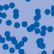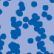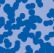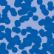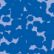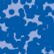## News

Florian Schweiger erhielt den Hausdorff-Gedächtnispreis 2021 der Fachgruppe Mathematik für die beste Dissertation. Er fertigte die Dissertation unter der Betreuung von Prof. Stefan Müller an. Unter anderen wurde Vanessa Ryborz mit einem Preis der Bonner Mathematischen Gesellschaft für ihre von Prof. Sergio Conti betreute Bachelorarbeit ausgezeichnet. (18.01.2022)

Prof. Dr. Sergio Albeverio has been elected into the Academia Europaea and the Accademia Nazionale dei Lincei (more; 02.12.2021).

Der SFB 1060 Die Mathematik der emergenten Effekte hat eine dritte Förderperiode erhalten. (26.11.20)

Prof. Dr. Andreas Eberle erhält den diesjährigen Lehrpreis der Universität Bonn. (22.07.2020)

Herr Dr. Richard Höfer erhielt den Hausdorff-Gedächtnispreis 2019 der Fachgruppe Mathematik für die beste Disseration. Betreut wurde die Arbeit von Prof. J. Velázquez (29.01.2020).

Contact

Managing Director: Prof. Dr. Massimiliano Gubinelli